NEET  >  NCERT Exemplar: Solutions

# NCERT Exemplar: Solutions - Notes | Study Chemistry Class 12 - NEET

 Table of contentsMultiple Choice Questions - IMultiple Choice Questions - IIShort Answer Type QuestionsMatching TypeAssertion and Reason TypeLong Answer Type Questions1 Crore+ students have signed up on EduRev. Have you?

Multiple Choice Questions - I

Q.1. Which of the following units is useful in relating concentration of solution with its vapour pressure?
(a) Mole fraction
(b) Parts per million
(c) Mass percentage
(d) Molality
Ans. (a)
Solution.
Mole fraction is useful in relating vapour pressure with concentration of solution. According to Raoult’s law, the partial vapour pressure of each component in the solution is directly proportional to its mole fraction.
A is one component.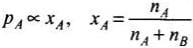Q.2. On dissolving sugar in water at room temperature solution feels cool to touch.
Under which of the following cases dissolution of sugar will be most rapid?
(a) Sugar crystals in cold water.
(b) Sugar crystals in hot water.
(c) Powdered sugar in cold water.
(d) Powdered sugar in hot water.

Ans. (d)
Solution.

Dissolution of sugar in water will be most rapid when powdered sugar is dissolved in hot water because powder form can easily insert in the vacancies of liquid particles. Further dissolution of sugar in water is an endothermic process. Hence, high temperature will favour the dissolution of sugar in water.

Q.3. At equilibrium the rate of dissolution of a solid solute in a volatile liquid  solvent is ______.
(a) Less than the rate of crystallisation
(b) Greater than the rate of crystallisation
(c) Equal to the rate of crystallisation
(d) Zero
Ans. (c)
Solution.

At equilibrium the rate of dissolution of solid in a volatile liquid solvent is equal to the rate of crystallisation.

Q.4. A beaker contains a solution of substance ‘A’. Precipitation of substance ‘A’ takes place when small amount of ‘A’ is added to the solution. The solution is ______.
(a) Saturated
(b) Supersaturated
(c) Unsaturated
(d) Concentrated

Ans. (b)
Solution.

When solute is added to the solution three cases may arise
(a) It dissolves in solution then solution is unsaturated.
(b) It does not dissolve in the solution then solution is known as saturated.
(c) When solute gets precipitated solution is known as supersaturated solution.

Q.5. Maximum amount of a solid solute that can be dissolved in a specified amount of a given liquid solvent does not depend upon ______.
(a) Temperature
(b) Nature of solute
(c) Pressure
(d) Nature of solvent
Ans. (c)
Solution.

Maximum amount of solid that can be dissolved in a specified amount of a given solvent does not depend upon pressure. This is because solid and liquid are highly incompressible and practically remain unaffected by change in pressure.

Q.6. Low concentration of oxygen in the blood and tissues of people living at high altitude is due to ______.
(a) Low temperature
(b) Low atmospheric pressure
(c) High atmospheric pressure
(d) Both low temperature and high atmospheric pressure
Ans. (b)
Solution.

Low concentration of oxygen in the blood and tissues of people living at high altitude is due to low atmospheric pressure. Because at high altitude, the partial, pressure of oxygen is less than at the ground level, so the decreased atmospheric pressure causes release of oxygen from blood.

Q.7. Considering the formation, breaking and strength of hydrogen bond, predict which of the following  mixtures will show a positive deviation from Raoult’s law?
(a) Methanol and acetone.
(b) Chloroform and acetone.
(c) Nitric acid and water.
(d) Phenol and aniline.
Ans. (a)
Solution.

In pure methanol, molecules are hydrogen bonded. On adding acetone, its molecules get in between the host molecules and break some of the hydrogen bonds between them.
Therefore, the intermolecular attractive forces between the solute-solvent molecules are weaker than those between the solute-solute and solvent-solvent molecules.
On the other hand, other three remaining options will show negative deviation from Raoult's law where the intermolecular attractive forces between the solute-solvent molecules are stronger than those between the solute-solute and solvent-solvent molecules.

Q.8. Colligative properties depend on ______.
(a) The nature of the solute particles dissolved in solution.
(b) The number of solute particles in solution.
(c) The physical properties of the solute particles dissolved in solution.
(d) The nature of solvent particles.
Ans. (b)
Solution.

Colligative properties depend upon number of solute particles in solution irrespective of their nature.

Q.9. Which of the following aqueous solutions should have the highest boiling point?
(a) 1.0 M NaOH
(b) 1.0 M Na2SO4
(c) 1.0 M NH4NO3
(d) 1.0 M KNO3
Ans. (b)
Solution.

As we know greater the value of van't Hoff factor higher will be the elevation in boiling point and hence higher will be the boiling point of solution.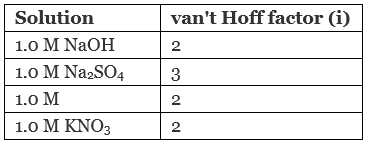Hence, 1.0 M Na2SO4 has highest value of boiling point.

Q.10. The unit of ebullioscopic constant is ______.
(a) K kg mol–1 or K (molality)–1
(b) mol kg K–1 or K–1(molality)
(c) kg mol–1 K–1 or K–1(molality)–1
(d) K mol kg–1 or K (molality)
Ans. (a)
Solution.

As we know from elevation in boiling point is given as:
ΔTb = Kbm
Kb = ΔTb/m
Where Kb = ebullioscopic constant
Thus, unit of K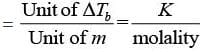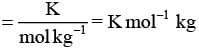Q.11. In comparison to a 0.01 M solution of glucose, the depression in freezing point of  a 0.01 M MgCl2 solution is ______.
(a) The same
Ans. (c)
Solution.

As we know depression in freezing point is directly related to van't Hoff factor (i) according to which greater the value of i greater will be the depression in freezing point.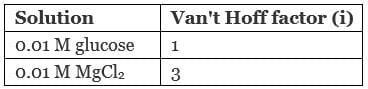Hence, depression in freezing point of glucose is about 3 times of glucose.

Q.12. An unripe mango placed in a concentrated salt solution to prepare pickle, shrivels because ______.
(a) It gains water due to osmosis.
(b) It loses water due to reverse osmosis.
(c) It gains water due to reverse osmosis.
(d) It loses water due to osmosis.
Ans. (d)
Solution.

When an unripe mango is placed in a concentrated salt solution to prepare pickle then mango loose water due to osmosis and get shrivel.

Q.13. At a given temperature, osmotic pressure of a concentrated solution of a substance ______.
(a) Is higher than that at a dilute solution.
(b) Is lower than that of a dilute solution.
(c) Is same as that of a dilute solution.
(d) Cannot be compared with osmotic pressure of dilute solution.
Ans. (a)
Solution.

According to definition of osmotic pressure we know that π = CRT. For concentrated solution C has higher value than dilute solution.
Hence, as concentration of solution increases osmotic pressure will also increase.

Q.14. Which of the following statements is false?
(a) Two different solutions of sucrose of same molality prepared in different solvents will have the same depression in freezing point.
(b) The osmotic pressure of a solution is given  by the equation π = CRT (where C is the molarity of the solution).
(c) Decreasing order of osmotic pressure for 0.01 M aqueous solutions of barium chloride, potassium chloride, acetic acid and sucrose is BaCl2 > KCl > CH3COOH > sucrose.
(d) According to Raoult’s law, the vapour pressure exerted by a volatile component of a solution is directly proportional to its mole fraction in the solution.
Ans. (a)
Solution.

Two different solutions of sucrose of same molality prepared in different solvents will have the same depression in freezing point.
According to definition of depression in freezing point.
ΔT= Kfm
Where, Kf = freezing point depression constant, value of Kf depends upon nature of solvent. This is why although the solutions have same molality two different solutions of sucrose of same molality prepared in different solvents will have different depression in freezing point.

Q.15. The values of Van’t Hoff factors for KCl, NaCl and K2SO4, respectively, are ______.
(a) 2, 2 and 2
(b) 2, 2 and 3
(c) 1, 1 and 2
(d) 1, 1 and 1

Ans. (b)
Solution.

Number of total ions present in the solution is known as van't Hoff factors (i).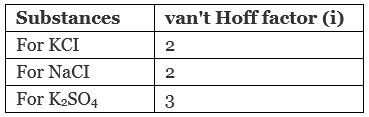Hence, correct choice is (ii).

Q.16. Which of the following statements is false?
(a) Units of atmospheric pressure and osmotic pressure are the same.
(b) In reverse osmosis, solvent molecules move through a semipermeable membrane from a region of lower concentration of solute to a region of higher concentration.
(c) The value of molal depression constant depends on nature of solvent.
(d) Relative lowering of vapour pressure, is a dimensionless quantity.
Ans. (b)
Solution.

In reverse osmosis, solvent molecules move through a semipermeable membrane from a region of higher concentration of solute to lower concentration.

Q.17. Value of Henry’s constant KH ______.
(a) Increases with increase in temperature.
(b) Decreases with increase in temperature.
(c) Remains constant.
(d) First increases then decreases.
Ans. (a)
Solution.

Value of Henry's constant (KH) increases with increase in temperature representing the decrease in solubility.

Q.18. The value of Henry’s constant KH is ______.
(a) Greater for gases with higher solubility.
(b) Greater for gases with lower solubility.
(c) Constant for all gases.
(d) Not related to the solubility of gases.
Ans. (b)
Solution.

According to Henry's law
p ∝ x
⇒ p = KHx
As value of KH rises solubility of gases decreases.

Q.19. Consider the Figure and mark the correct option.
(a) Water will move from side (A) to side (B) if a pressure lower than osmotic pressure is applied on piston (B).
(b) Water will move from side (B) to side (A) if a pressure greater than osmotic pressure is applied on piston (B).
(c) Water will move from side (B) to side (A) if a pressure equal to osmotic pressure is applied on piston (B).
(d) Water will move from side (A) to side (B) if pressure equal to osmotic pressure is applied on piston (A).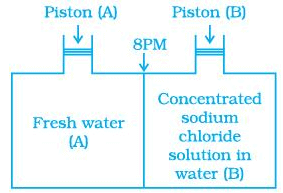Ans. (b)
Solution.
We know that, if a pressure higher than the osmotic pressure is applied on the solution. the solvent will flow from the solution into the pure solvent through the semi-permeable membrane. This process is called reverse osmosis.
Thus, in this case, water will move from side (B) to side (A) if a pressure greater than osmotic pressure is applied on piston (B).

Q.20. We have three aqueous solutions of NaCl labelled as ‘A’, ‘B’ and ‘C’ with concentrations 0.1M, 0.01M and 0.001M, respectively. The value of van’t Hoff factor for these solutions will be in the order ______.
(a) iA < iB < iC
(b) iA > iB > iC
(c) iA = iB = iC
(d) iA < iB > iC

Ans. (b)
Solution.

Van't Hoff factor is the measurement of total number of ions present in the solution. Therefore, greater the concentration of solution greater will be its van't Hoff factor.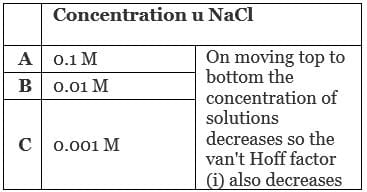Q.21. On the basis of information given below mark the correct option.
Information:
(i) In bromoethane and chloroethane mixture intermolecular interactions of A–A and B–B type are nearly same as A–B type interactions.
(ii) In ethanol and acetone mixture A–A or B–B type intermolecular interactions are stronger than A–B type interactions.
(iii) In chloroform and acetone mixture A–A or B–B type intermolecular interactions are weaker than A–B type interactions.
(a) Solution (B) and (C) will follow Raoult’s law.
(b) Solution (A) will follow Raoult’s law.
(c) Solution (B) will show negative deviation from Raoult’s law.
(d) Solution (C) will show positive deviation from Raoult’s law.

Ans. (b)
Solution.

For an ideal solution, the A—A or B—B type intermolecular interaction is nearly equal to A—B type interaction. Here, a mixture of bromoethane and chloroethane is an example of ideal solution.
On the other hand chloroform and acetone mixture is an example of non-ideal solution having negative deviation. So, (A — A) or (B—B) interaction must be stronger than A—B interaction. While ethanol-acetone mixture shows positive deviation due to weaker A—B interaction in comparison to A—A or A—B interaction.

Q.22. Two beakers of capacity 500 mL were taken. One of these beakers, labelled as “A”, was filled with 400 mL water whereas the beaker labelled “B” was filled with 400 mL of 2M solution of NaCl. At the same temperature both the beakers were placed in closed containers of same material and same capacity as shown in Figure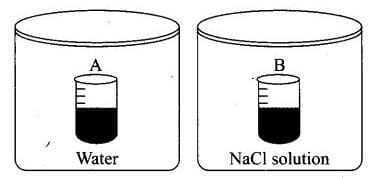At a given temperature, which of the following statement is correct about the vapour pressure of pure water and that of NaCl solution.
(a) Vapour pressure in container (A) is more than that in container (B).
(b) Vapour pressure in container (A) is less than that in container (B).
(c) Vapour pressure is equal in both the containers.
(d) Vapour pressure in container (B) is twice the vapour pressure in container (A).

Ans. (a)
Solution.

When salt is added to water to make the solution, the vapour pressure of solution decreases. This is due to decrease in surface covered by solvent molecule which leads to decrease in number of solvent molecule escaping from the surface corresponding to pure solvent.
Hence, vapour pressure also get reduces.

Q.23. If two liquids A and B form minimum boiling azeotrope at some specific composition then ________.
(a) A–B interactions are stronger than those between A–A or B–B.
(b) Vapour pressure of solution increases because more number of molecules of liquids A and B can escape from the solution.
(c) Vapour pressure of solution decreases because less number of molecules of only one of the liquids escape from the solution.
(d) A–B interactions are weaker than those between A–A or B–B.
Ans. (a)
Solution.

If two liquids A and B form minimum boiling azeotrope at some specific composition then A—B interactions are weaker than those of A—A and B—B. It is due to the fact that in case of positive deviation, we get minimum boiling azeotropes whereas in case of negative deviation we get maximum boiling azeotropes.

Q.24. 4L of 0.02 M aqueous solution of NaCl was diluted by adding one litre of water. The molality of the resultant solution is ______.
(a) 0.004
(b) 0.008
(c) 0.012
(d) 0.016

Ans. (d)
Solution.

Given, M1 = 0.02M, V1 = 4L, M2 = ?, V2 = 4L + 1L = 5L
As we know, M1V1 = M2V2
Where, V1 = volume of solution before dilution
V2 = volume of solution after dilution
M= strength of solution before dilution
M2 = strength of solution after dilution
0.02 × 4L = M2 × 5L
M2 = 0.08/5 = 0.016 M

Q.25. On the basis of information given below mark the correct option.
Information: On adding acetone to methanol some of the hydrogen bonds between methanol molecules break.
(a) At specific composition methanol-acetone mixture will form minimum boiling azeotrope and will show positive deviation from Raoult’s law.
(b) At specific composition methanol-acetone mixture will forms maximum boiling azeotrope and will show positive deviation from Raoult’s law.
(c) At specific composition methanol-acetone mixture will form minimum boiling azeotrope and will show negative deviation from Raoult’s law.
(d) At specific composition methanol-acetone mixture will form maximum boiling azeotrope and will show negative deviation from Raoult’s law.

Ans. (a)
Solution.

At specific composition methanol- acetone mixture will form minimum boiling azeotrope and will show positive deviation. This is due to weaker A—B interaction than A—A or B—B interaction. i.e., A—B < A—A and B—B

Q.26. KH value for Ar(g), CO2(g), HCHO (g) and CH4(g) are 40.39, 1.67, 1.83×10–5 and 0.413 respectively.
Arrange these gases in the order of their increasing solubility.
(a) HCHO < CH4  < CO2 < Ar
(b) HCHO < CO2 < CH4 < Ar
(c) Ar < CO2 < CH4 < HCHO
(d) Ar < CH< CO2 < HCHO

Ans. (c)
Solution.

We know that higher the value of KH at a given pressure, the lower is the solubility of the gas in the liquid.
Now, value of KH depends upon nature of gases dissolved in water.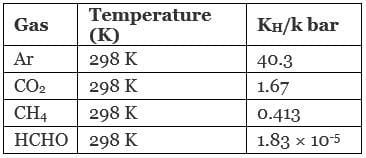Hence, correct order is Ar < CO2 < CH4 < HCHO and correct choice is (iii).

Multiple Choice Questions - II
Note: In the following questions two or more options may be correct.
Q.27. Which of the following factor (s) affect the solubility of a gaseous solute in the fixed volume of liquid solvent?
(a) Nature of solute
(b) Temperature
(c) Pressure
(i) (a) and (c) at constant T
(ii) (a) and (b) at constant P
(iii) (b) and (c) only
(iv) (c) only

Ans. (i, ii)
Solution.

Solubility of gaseous solute in the fixed volume of liquid solvent always depends upon nature of solute. It also depends upon pressure at constant temperature and temperature at constant pressure.

Q.28. Intermolecular forces between two benzene molecules are nearly of same strength as those between two toluene molecules. For a mixture of benzene and toluene, which of the following are not true?
(a) ∆mix H = zero
(b) ∆mix V = zero
(c) These will form minimum boiling azeotrope.
(d) These will not form ideal solution.
Ans. (c, d)
Solution.

The solution which follows Raoult's law is known as ideal solution. For an ideal solution intermolecular forces between two benzene molecules are nearly of same strength as those between two toluene molecules. For an ideal solution
∆Vmix = 0 and ∆Hmix = 0
Thus, the mixture of benzene and toluene is an example of ideal solution. Option (iii) is incorrect as minimum boiling azeotropes are formed by non-ideal solution.

Q.29. Relative lowering of vapour pressure is a colligative property because ______.
(a) It depends on the concentration of a non electrolyte solute in solution and does not depend on the nature of the solute molecules.
(b) It depends on number of particles of electrolyte solute in solution and does not depend on the nature of the solute particles.
(c) It depends on the concentration of a non electrolyte solute in solution as well as on the nature of the solute molecules.
(d) It depends on the concentration of an electrolyte or nonelectrolyte solute in  solution as well as on the nature of solute molecules.

Ans. (a, b)
Solution.

Relative lowering of vapour pressure is a Colligative property because
(a) It does not depend upon nature of solute.
(b) It depends upon number of solute particles.
(c) It depends upon concentration of non-electrolyte solution.

Q.30. Van’t Hoff factor (i) is given by the expression ______.
(a)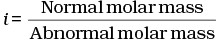(b)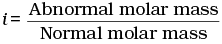(c)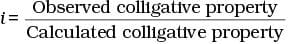(d)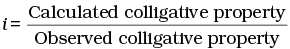Ans. (a, c)
Solution.

Van't Hoff factor (i) is a measure of extent of association or dissociation of solute particles which can be calculated as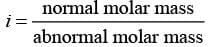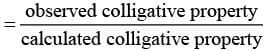Q.31. Isotonic solutions must have the same ______.
(a) Solute
(b) Density
(c) Elevation in boiling point
(d) Depression in freezing point
Ans. (c, d)
Solution.

Isotonic solutions have same osmotic pressure and same concentration. Elevation in boiling point and depression in freezing point are the Colligative properties. These two Colligative properties depend upon concentration.
As the molar concentration is same for isotonic solutions, so elevation in boiling point and depression in freezing point of isotonic solutions must be same.

Q.32. Which of the following binary mixtures will have same composition in liquid and vapour phase?
(a) Benzene - Toluene
(b) Water -Nitric acid
(c) Water -Ethanol
(d) n-Hexane - n-Heptane
Ans. (b, c)
Solution.

Mixtures having same composition in liquid and vapour phase are known as azeotropes. Azeotrope boils at same temperature.
Here, water-nitric acid and water-ethanol mixtures are non-ideal solution. Hence, water-nitric acid and water-ethanol are examples of azeotropes.
While benzene-toluene and n-hexane-n -heptane are examples of ideal solution.

Q.33. In isotonic solutions ______.
(a) Solute and solvent both are same.
(b) Osmotic pressure is same.
(c) Solute and solvent may or may not be same.
(d) Solute is always same solvent may be different.
Ans. (b, c)
Solution.

The two solutions having same osmotic pressure are known as isotonic solutions. The solute and solvent particles may or may not be same but osmotic pressure must be same.

Q.34. For a binary ideal liquid solution, the variation in total vapour pressure versus composition of solution is given by which of the curves?
(a)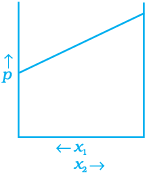(b)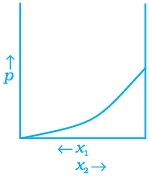(c)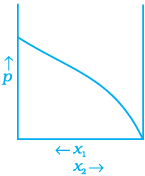(c)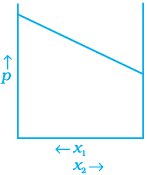Ans. (a, d)
Solution.
Depending on the vapour pressures of the pure components 1 and 2, total vapour pressure over the solution decreases or increases with the increase of the mole fraction of component 1.

Q.35. Colligative properties are observed when ______.
(a) A non volatile solid is dissolved in a volatile liquid.
(b) A non volatile liquid is dissolved in another volatile liquid.
(c) A gas is dissolved in non volatile liquid.
(d) A volatile liquid is dissolved in another volatile liquid.

Ans. (a, b)
Solution.

When any of one components of binary mixture either solvent or solute is volatile it causes deviation from ideal behaviour and vapour pressure of solution which causes change in Colligative property.

Q.36. Components of a binary mixture of two liquids A and B were being separated by distillation. After some time separation of components stopped and composition of vapour phase became same as that of liquid phase. Both the components started coming in the distillate. Explain why this happened.
Ans. Both the components are appearing in the distillate and composition of liquid and vapour is same. This shows that liquids have formed azeotropic mixture and boil at constant temperature hence cannot be separated at this stage by distillation or fractional distillation. Solution having azeotropic nature shows large positive or negative deviation from Raoult's law depending upon intermolecular interaction.

Q.37. Explain why on addition of 1 mol of NaCl to 1 litre of water, the boiling point of water increases, while addition of 1 mol of methyl alcohol to one litre of water decreases its boiling point.
Ans. NaCI is a non-volatile solute. So, its addition to water lowers the vapour pressure of the water. Hence, boiling point of water (solution) increases. On the other hand, methyl alcohol is more volatile than water.
So, its addition to water increases the total vapour pressure over the solution. It results in the decrease of boiling point.

Q.38. Explain the solubility rule “like dissolves like” in terms of intermolecular forces that exist in solutions.
Ans. If the intermolecular interactions are similar in both constituents, i.e., solute and solvent then solute dissolves in the solvent.
Example: polar solutes dissolve in polar solvents and non-polar solutes in non-polar solvents.
Thus, the statement 'like dissolves like' proves to be true.
Example: organic compounds dissolve in non-polar organic solvent while polar inorganic compounds (salts) dissolve in polar solvent (water).

Q.39. Concentration terms such as mass percentage, ppm, mole fraction and molality are independent of temperature, however molarity is a function of temperature. Explain.
Ans. Molarity is defined as the number of moles of solute dissolved per litre of a solution. Since, volume depends on temperature and changes with change in temperature, the molarity will also change with change in temperature.
On the other hand, mass does not change with change in temperature, so other concentration terms given in the question also do not do so. Thus, temperature has no effect on the mass but it has significant effect on volume.

Q.40. What is the significance of Henry’s Law constant KH?
Ans. According to Henry's law
p ∝ x ⇒ p = KHx
Higher the value of Henry's law constant KH, the lower is the solubility of the gas in a liquid. Thus, the solubility of a gas in the given liquid can be increased by increasing pressure.

Q.41. Why are aquatic species more comfortable in cold water in comparison to warm water?
Ans. Aquatic species are more comfortable in cold water due to the presence of more oxygen. Solubility of oxygen in water increases with decrease in temperature as solubility of a gas in given liquid decreases with increase in temperature.

Q.42.
(a) Explain the following phenomena with the help of Henry’s law.
(i) Painful condition known as bends.
(ii) Feeling of weakness and discomfort in breathing at high altitude.
(b) Why soda water bottle kept at room temperature fizzes on opening?
Ans.
(a)
(i) Henry's law represents a relation between solubility of gases in liquid and pressure. Scuba drivers when come towards surface, the pressure gradually decreases. This reduced pressure releases the dissolved gas present in blood and leads to formation of bubbles of nitrogen in the blood. This creates a painful condition by blocking capillaries known as blends.
(ii) At high altitude atmospheric pressure is low as compared to surface which causes difficulty in breathing. In that condition we feel weakness and discomfort.
(b) Soda water bottle kept at room temperature fizzes on opening due to different pressure inside and outside the bottle. When the bottle is opened to air, the partial pressure of CO2 is above the solution decreases. As a result, solubility decreases and hence CO2 bubbles out with a fizz.

Q.43. Why is the vapour pressure of an aqueous solution of glucose lower than that of water?
Ans. In pure liquid, the entire surface of liquid is occupied by the molecules of water. When a non-volatile solute,
Example: glucose is dissolved in water.
the fraction of surface covered by the solvent molecules gets reduced because some positions are occupied by glucose molecules.
So, number of solvent molecules escaping from the surface is reduced. That is why vapour pressure of aqueous solution of glucose is reduced.

Q.44. How does sprinkling of salt help in clearing the snow covered roads in hilly areas? Explain the phenomenon involved in the process.
Ans. When salt is spread over snow covered roads, snow starts melting from the surface due to the depression in freezing point of water on addition of a non-volatile solute (salt) to it. It helps in clearing of roads.
Hence, the phenomenon involved is ‘depression in freezing point of water when a non volatile solute is dissolves in it.’

Q.45. What is “semi permeable membrane”?
Ans. Continuous sheets or films (natural or synthetic) which contain a network of submicroscopic holes or pores through which small solvent molecules like water can pass; but the passage of bigger molecules of solute is hindered, are known as semi permeable membrane.
Example: cellophane membrane.

Q.46. Give an example of a material used for making semipermeable membrane for carrying out reverse osmosis.
Ans. Since pressure required for the reverse osmosis is very high, so, a suitable material is used for making semipermeable membrane. It is generally cellulose acetate placed over suitable support.

Matching Type

Note : In the following questions match the items given in Column I and Column II.
Q.47. Match the items given in Column I and Column II.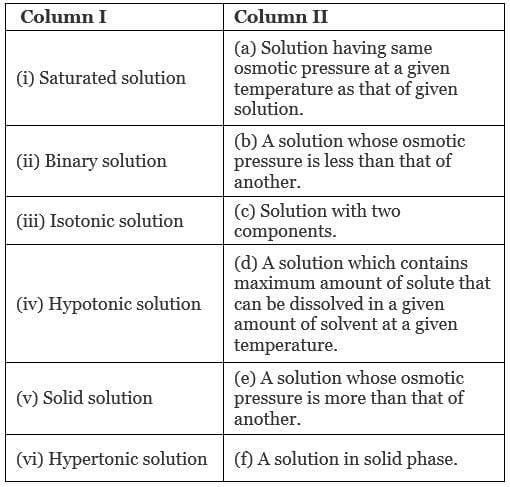Ans. (i → d), (ii → c); (iii → a); (iv → b), (v → f); (vi → e)
Solution.
(i) Saturated solution: A solution which contains maximum amounts of solute that can be dissolved in a given amount of solvent at a given temperature.
(ii) Binary solution: A solution with two components is known as binary solution.
(iii) Isotonic solution: A solution having same osmotic pressure at a given temperature as that of given solution is known as isotonic solution.
(iv) Hypotonic solution: A solution whose osmotic pressure is less than another is known as hypotonic solution.
(v) Solid solution: A solution in solid phase is known as solid solution.
(vi) Hypertonic solution: A solution whose osmotic pressure is greater than that of another is known as hypertonic solution.

Q.48. Match the items given in Column I with the type of solutions given in Column II.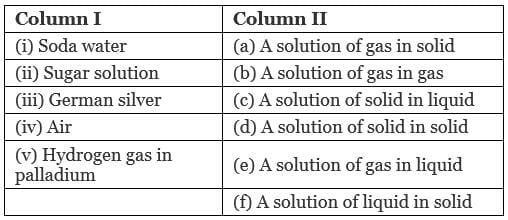Ans. (i → e), (ii → c), (iii → d); (iv → b), (v → a)
Solution.
(i) Soda water: A solution of gas in liquid.
Example: CO2 in soft drinks.
(ii) Sugar solution: A solution of solid in liquid in which sugar particles (soild) are dissolved in water (liquid).
(iii) German silver: German silver is an alloy which is a solid solution of solid in solid. It is an alloy of Cu, Zn and Ni.
(iv) Air: A solution of gas in gas. Air is a mixture of various gases.
(v) Hydrogen gas in palladium: This solution is an example of solution of gas in solid. This is used as an reducing agent.

Q.49. Match the laws given in Column I with expressions given in Column II.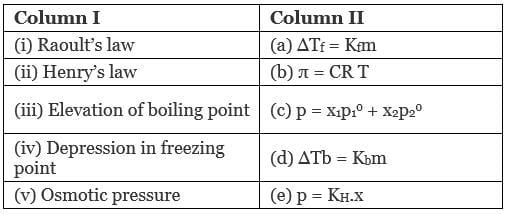Ans.  (i → c), (ii → e), (iii → a); (iv → d), (v → b)
Solution.
(a) Raoult's law: Mathematical representation of Raoult's law p = x1p10 + x2p02
(b) Henry's law: p = KH.x
(c) Elevation of boiling point: Mathematical representation, ∆Tb = Kbm
(d) Depression in freezing point: Mathematical representation, ∆Tf = Kfm
(e) Osmotic pressure: Mathematical representation, π = CRT

Q.50. Match the terms given in Column I with expressions given in Column II.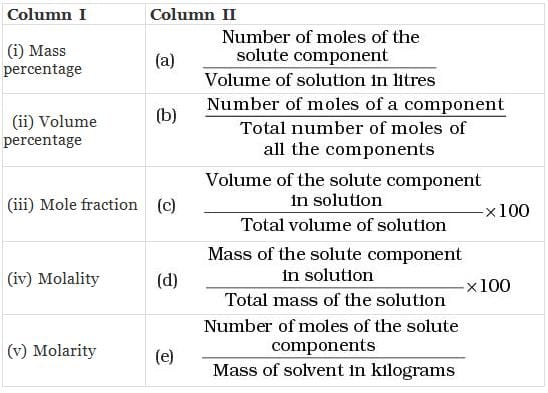Ans. (i → d), (ii →  c), (iii →  b), (iv →  e), (v → a)
Solution.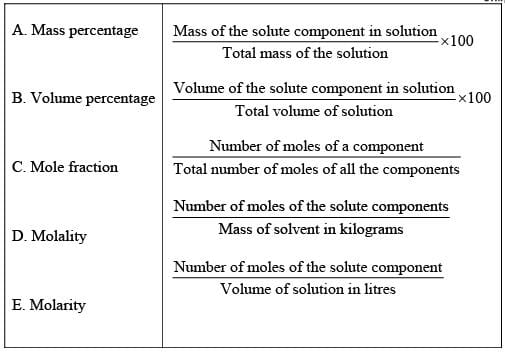Assertion and Reason Type

Note : In the following questions a statement of assertion followed by a statement of reason is given. Choose the correct answer out of the following choices.

Q.51. Assertion: Molarity of a solution in liquid state changes with temperature.

Reason: The volume of a solution changes with change in temperature.

(i) Assertion and reason both are correct statements and reason is correct explanation for assertion.
(ii) Assertion and reason both are correct statements but reason is not correct explanation for assertion.
(iii) Assertion is correct statement but reason is wrong statement.
(iv) Assertion and reason both are incorrect statements.
(v) Assertion is wrong statement but reason is correct statement.

Ans. (i)
Solution.

Volume of solutions is a function of temperature which varies with temperature. Hence, molarity of solution in liquid state changes with temperature.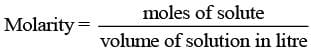Q.52. Assertion : When methyl alcohol is added to water, boiling point of water increases.

Reason : When a volatile solute is added to a volatile solvent elevation in boiling point is observed.

(i) Assertion and reason both are correct statements and reason is correct explanation for assertion.
(ii) Assertion and reason both are correct statements but reason is not correct explanation for assertion.
(iii) Assertion is correct statement but reason is wrong statement.
(iv) Assertion and reason both are incorrect statements.
(v) Assertion is wrong statement but reason is correct statement.

Ans. (v)
Solution.

When methyl alcohol is added to water, boiling point of water decreases because when a volatile solute is added to a volatile solvent elevation in boiling point is observed.

Q.53. Assertion : When NaCl is added to water a depression in freezing point is observed.

Reason : The lowering of vapour pressure of a solution causes depression in the freezing point.

(i) Assertion and reason both are correct statements and reason is correct explanation for assertion.
(ii) Assertion and reason both are correct statements but reason is not correct explanation for assertion.
(iii) Assertion is correct statement but reason is wrong statement.
(iv) Assertion and reason both are incorrect statements.
(v) Assertion is wrong statement but reason is correct statement.

Ans. (i)
Solution.

When NaCl is added to water a depression in freezing point is observed. This is due to lowering of vapour pressure of a solution. Lowering of vapour pressure is observed due to intermolecular interaction of solvent-solute particles.

Q.54. Assertion: When a solution is separated from the pure solvent by a semipermeable membrane, the solvent molecules pass through it from pure solvent side to the solution side.

Reason: Diffusion of solvent occurs from a region of high concentration solution to a region of low concentration solution.

(i) Assertion and reason both are correct statements and reason is correct explanation for assertion.
(ii) Assertion and reason both are correct statements but reason is not correct explanation for assertion.
(iii) Assertion is correct statement but reason is wrong statement.
(iv) Assertion and reason both are incorrect statements.
(v) Assertion is wrong statement but reason is correct statement.

Ans. (ii)
Solution.

When a solution is separated from the pure solvent by a semipermeable membrane, the solvent molecules pass through it from pure solvent side to the solution side. Solvent molecules always flow from lower concentration to higher concentration of solution.

Q.55. Define the following modes of expressing the concentration of a solution. Which of these modes are independent of temperature and why?
(i) w/w (mass percentage)
(ii) V/V (volume percentage)
(iii) w/V (mass by volume percentage)
(iv) ppm. (parts per million)
(v) x (mole fraction)
(vi) M (Molarity)
(vii) m (Molality)
Ans.
(i) w/w (mass percentage): Mass percentage of a component of a solution can be expressed as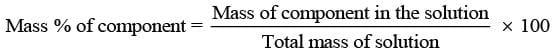Thus, the percentage by mass means the mass of the solute in grams present in 100g of the solution.
(ii) V/V (volume percentage) is defined as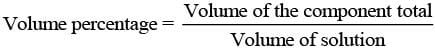Thus, volume percentage means the volume of the liquid solute in cm3 present in 100 cm3 of the solution.
(iii) w/V (mass by volume percentage) = mass of solute dissolved in 100 mL of solution.
(iv) ppm (parts per million): This parameter is used to express the concentration of very dilute solution.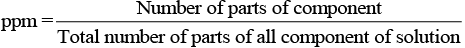(v) x (mole fraction): Mole fraction is a unitless quantity used to determine extent of any particular component present in total solution.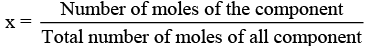(vi) M (molarity): Number of moles of solute dissolved in per litre of solution is known as molarity.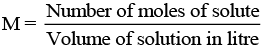(vii) m (Molality): Molality of any solution can be defined as number of moles of solute dissolved in per kg of solvent.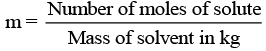Q.56. Using Raoult’s law explain how the total vapour pressure over the solution is related to mole fraction of components in the following solutions.
(i) CHCl3 (l) and CH2Cl2(l)
(ii) NaCl(s) and H2O (l)
Ans. According to Raoult's law for any solution the partial vapour pressure of each volatile component in the solution is directly proportional to its mole fraction.
p1 = P°1x1
(a) CHCl3 (l) and CH2Cl2 (l) both are volatile components.
Hence, for a binary solution in which both components are volatile liquids, the total pressure will be
p = p1 + p2 = x1p°1 + x22
= x11 + (1-x1)p°2
=(p°1 - p°2)x1 + p°2
Where, p = total vapour pressure
p1 = partial vapour pressure of component 1
p2 = partial vapour pressure of component 2
(b) NaCl (s) and H2O (l) both are non-volatile components. Hence, for a solution containing nonvolatile solute, the Raoult's law is applicable only to vaporisable component and total vapour pressure can be written as
p = p1 = x11
where p°1 reresents the vapour pressure of pure H2O(l).

Q.57. Explain the terms ideal and non-ideal solutions in the light of forces of interactions operating between molecules in liquid solutions.
Ans. The solutions which obey Raoult's law over the entire range of concentration are known as ideal solutions. In case of an ideal solution, enthalpy of mixing, ΔHmix = 0 and volume change on mixing, ΔVmix = 0. The ideal behaviour of the solutions can be explained by considering two components A and B.
In pure components, the intermolecular attractive interactions will be of A—A type and B—B type, whereas in the binary solutions in addition to these two, A—B type of interaction will also be present. If A—A and B—B intermolecular forces are nearly equal to those between A—B, this leads to the formation of ideal solution.
Example: solution of n-hexane and n-heptane.
When a solution does not obey-Raoult's law over the entire range of concentration, then it is called nonideal solution. The vapour pressure of such a solution is either higher or lower, than that predicted by Raoult's law.
If it is higher, the solution exhibits positive deviation and if it is lower it exhibits negative deviation from Raoult's law. In case of positive deviation, A—B interactions are weaker than those between A—A or B—B. i.e., the attractive forces between solute solvent molecules are weaker than those between solute-solute and solvent-solvent molecules.
Example: mixture of ethanol and acetone.
For such solutions ΔHmixing = + ve and ΔVmixing = + ve
On the other hand, in case of negative deviation the intermolecular attractive forces between A—A and B—B are weaker than those between A—B molecules. Thus, the escaping tendency of A and B types of molecules from the solution becomes less than from the pure liquids i.e., mixture of chloroform and acetone.
For such solutions ΔHmix = - ve and ΔVmix = - ve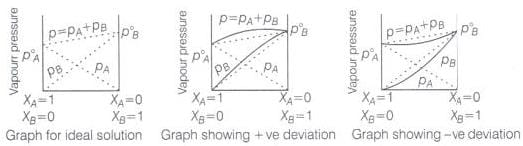Q.58. Why is it not possible to obtain pure ethanol by fractional distillation? What general name is given to binary mixtures which show deviation from Raoult’s law and whose components cannot be separated by fractional distillation. How many types of such mixtures are there?
Ans. The solution or mixture having same composition in liquid as well as in vapour phase and boils at a constant temperature is known as azeotropes. Due to constant composition it can't be separated by fractional distillation. There are two types of azeotropes
(a) Minimum boiling azeotropes: Solutions which show large positive deviation from Raoult's law form minimum boiling azeotropes at a specific composition.
Example: ethanol —water mixture
(b) Maximum boiling azeotropes: Solutions which show large negative deviation from Raoult's law form maximum boiling azeotropes.
Example: solution having composition 68% HNO3 and 32% water by mass.

Q.59. When kept in water, raisin swells in size. Name and explain the phenomenon involved with the help of a diagram. Give three applications of the phenomenon.

Ans. This phenomenon is called endo osmosis, i.e., movement of water inside the raisin and shown with the help of diagram as the process of osmosis is of immense biological as well as industrially important. It is evident from the following examples.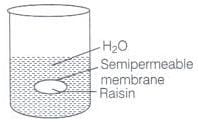(i) Movement of water from soil into plant roots and subsequently into upper portion of the plant is partly due to osmosis.
(ii) Preservation of meat against bacterial action by addition of salt.
(iii) Preservation of fruits against bacterial action by adding sugar. Bacterium in canned fruit loses water through the process of osmosis and become inactive.
(iv) Reverse-osmosis is used in desalination of water.

Q.60. Discuss biological and industrial importance of osmosis.
Ans.
(i) In animals, water moves into different parts of the body under the effect of the process of osmosis.
(ii) Stretching of leaves, flower, etc., is also controlled by osmosis.
(iii) Osmosis helps in rapid growth of the plants and germination of seeds.
(iv) Different movements of plants such as opening and closing of flowers etc, are controlled by osmosis.

Q.61. How can you remove the hard calcium carbonate layer of the egg without damaging its semipermeable membrane? Can this egg be inserted into a bottle with a narrow neck without distorting its shape? Explain the process involved.
Ans. When egg is placed in mineral acid solution outer shell of egg dissolves.
Egg is now removed and placed in hypertonic solution. Size of egg gets reduced and egg shrivels due to osmosis. Egg is now placed in a bottle with narrow neck. Finally on adding hypotonic solution egg regains its shape due to osmosis.
Diagrammatically it can be represented as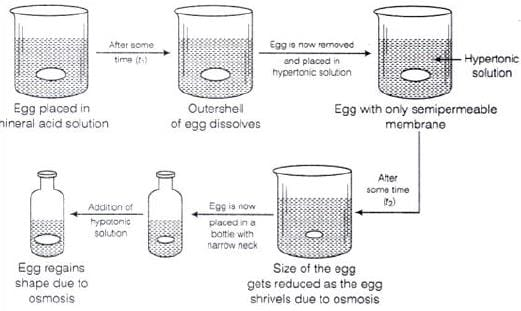Q.62. Why is the mass determined by measuring a colligative property in case of some solutes abnormal ? Discuss it with the help of Van’t Hoff factor.

Ans. Certain compounds when dissolved in suitable solvents either dissociate or associate.
Example: ethanoic acid dimerises in benzene due to H-bonding.
while in water, it dissociates and forms ions. As a result, the number of chemical species in solution increases or decreases as compared to the number of chemical species of solute added to form the solution.
Since, the magnitude of colligative property depends on the number of solute particles, it is expected that the molar mass determined on the basis of colligative properties will either higher or lower than the expected value or the normal value and is called abnormal molar mass.
In order to account for the extent of dissociation or association of molecules in solution, van't Hoff introduced a factor, i, known as the van't Hoff factor.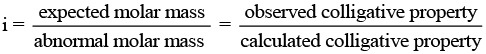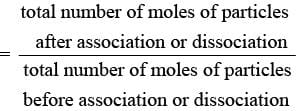The document NCERT Exemplar: Solutions - Notes | Study Chemistry Class 12 - NEET is a part of the NEET Course Chemistry Class 12.
All you need of NEET at this link: NEET

## Chemistry Class 12

157 videos|385 docs|261 tests
 Use Code STAYHOME200 and get INR 200 additional OFF

## Chemistry Class 12

157 videos|385 docs|261 tests

### How to Prepare for NEET

Read our guide to prepare for NEET which is created by Toppers & the best Teachers

Track your progress, build streaks, highlight & save important lessons and more!

,

,

,

,

,

,

,

,

,

,

,

,

,

,

,

,

,

,

,

,

,

;